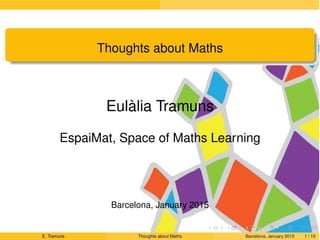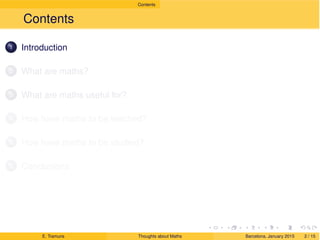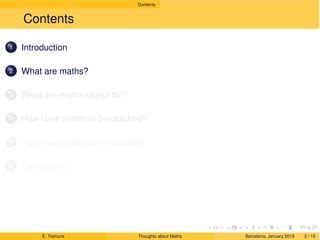Successfully reported this slideshow.×

# Maths thoughts

What are maths? Maths are everywhere and are useful for life. How have maths to be teached? How have maths to be learned? A brief summary of what I think is at this presentation.

What are maths? Maths are everywhere and are useful for life. How have maths to be teached? How have maths to be learned? A brief summary of what I think is at this presentation.

### Maths thoughts

1. 1. backgroundpage Draft Thoughts about Maths Eulàlia Tramuns EspaiMat, Space of Maths Learning Barcelona, January 2015 E. Tramuns Thoughts about Maths Barcelona, January 2015 1 / 15
2. 2. Draft Contents Contents 1 Introduction 2 What are maths? 3 What are maths useful for? 4 How have maths to be teached? 5 How have maths to be studied? 6 Conclusions E. Tramuns Thoughts about Maths Barcelona, January 2015 2 / 15
3. 3. Draft Contents Contents 1 Introduction 2 What are maths? 3 What are maths useful for? 4 How have maths to be teached? 5 How have maths to be studied? 6 Conclusions E. Tramuns Thoughts about Maths Barcelona, January 2015 2 / 15
4. 4. Draft Contents Contents 1 Introduction 2 What are maths? 3 What are maths useful for? 4 How have maths to be teached? 5 How have maths to be studied? 6 Conclusions E. Tramuns Thoughts about Maths Barcelona, January 2015 2 / 15
5. 5. Draft Contents Contents 1 Introduction 2 What are maths? 3 What are maths useful for? 4 How have maths to be teached? 5 How have maths to be studied? 6 Conclusions E. Tramuns Thoughts about Maths Barcelona, January 2015 2 / 15
6. 6. Draft Contents Contents 1 Introduction 2 What are maths? 3 What are maths useful for? 4 How have maths to be teached? 5 How have maths to be studied? 6 Conclusions E. Tramuns Thoughts about Maths Barcelona, January 2015 2 / 15
7. 7. Draft Contents Contents 1 Introduction 2 What are maths? 3 What are maths useful for? 4 How have maths to be teached? 5 How have maths to be studied? 6 Conclusions E. Tramuns Thoughts about Maths Barcelona, January 2015 2 / 15
8. 8. Draft Introduction 1 Introduction 2 What are maths? 3 What are maths useful for? 4 How have maths to be teached? 5 How have maths to be studied? 6 Conclusions E. Tramuns Thoughts about Maths Barcelona, January 2015 3 / 15
9. 9. Draft Introduction Introduction Everyone knows that Maths are important. E. Tramuns Thoughts about Maths Barcelona, January 2015 4 / 15
10. 10. Draft Introduction Introduction Everyone knows that Maths are important. But do we know what maths are? E. Tramuns Thoughts about Maths Barcelona, January 2015 4 / 15
11. 11. Draft Introduction Introduction Everyone knows that Maths are important. But do we know what maths are? Can maths help us to live better? E. Tramuns Thoughts about Maths Barcelona, January 2015 4 / 15
12. 12. Draft Introduction Introduction Everyone knows that Maths are important. But do we know what maths are? Can maths help us to live better? How can be maths teached in another way? E. Tramuns Thoughts about Maths Barcelona, January 2015 4 / 15
13. 13. Draft Introduction Introduction Everyone knows that Maths are important. But do we know what maths are? Can maths help us to live better? How can be maths teached in another way? How can be maths studied to get good results? E. Tramuns Thoughts about Maths Barcelona, January 2015 4 / 15
14. 14. Draft What are maths? 1 Introduction 2 What are maths? 3 What are maths useful for? 4 How have maths to be teached? 5 How have maths to be studied? 6 Conclusions E. Tramuns Thoughts about Maths Barcelona, January 2015 5 / 15
15. 15. Draft What are maths? What are maths? E. Tramuns Thoughts about Maths Barcelona, January 2015 6 / 15
16. 16. Draft What are maths? What are maths? E. Tramuns Thoughts about Maths Barcelona, January 2015 6 / 15
17. 17. Draft What are maths? What are maths? MATHS? E. Tramuns Thoughts about Maths Barcelona, January 2015 6 / 15
18. 18. Draft What are maths? What are maths? E. Tramuns Thoughts about Maths Barcelona, January 2015 6 / 15
19. 19. Draft What are maths? What are maths? MATHS? E. Tramuns Thoughts about Maths Barcelona, January 2015 6 / 15
20. 20. Draft What are maths? What are maths? E. Tramuns Thoughts about Maths Barcelona, January 2015 6 / 15
21. 21. Draft What are maths? What are maths? MATHS? E. Tramuns Thoughts about Maths Barcelona, January 2015 6 / 15
22. 22. Draft What are maths? What are maths? E. Tramuns Thoughts about Maths Barcelona, January 2015 6 / 15
23. 23. Draft What are maths? What are maths? MATHS? E. Tramuns Thoughts about Maths Barcelona, January 2015 6 / 15
24. 24. Draft What are maths? What are maths? YES!!!!, ALL OF THESE ARE MATHS E. Tramuns Thoughts about Maths Barcelona, January 2015 6 / 15
25. 25. Draft What are maths? What are maths? YES!!!!, ALL OF THESE ARE MATHS MATHS ARE EVERYWHERE!!!! E. Tramuns Thoughts about Maths Barcelona, January 2015 6 / 15
26. 26. Draft What are maths useful for? 1 Introduction 2 What are maths? 3 What are maths useful for? 4 How have maths to be teached? 5 How have maths to be studied? 6 Conclusions E. Tramuns Thoughts about Maths Barcelona, January 2015 7 / 15
27. 27. Draft What are maths useful for? What are maths useful for? E. Tramuns Thoughts about Maths Barcelona, January 2015 8 / 15
28. 28. Draft What are maths useful for? What are maths useful for? E. Tramuns Thoughts about Maths Barcelona, January 2015 8 / 15
29. 29. Draft What are maths useful for? What are maths useful for? E. Tramuns Thoughts about Maths Barcelona, January 2015 8 / 15
30. 30. Draft What are maths useful for? What are maths useful for? E. Tramuns Thoughts about Maths Barcelona, January 2015 8 / 15
31. 31. Draft What are maths useful for? What are maths useful for? E. Tramuns Thoughts about Maths Barcelona, January 2015 8 / 15
32. 32. Draft What are maths useful for? What are maths useful for? E. Tramuns Thoughts about Maths Barcelona, January 2015 8 / 15
33. 33. Draft What are maths useful for? What are maths useful for? E. Tramuns Thoughts about Maths Barcelona, January 2015 8 / 15
34. 34. Draft What are maths useful for? What are maths useful for? E. Tramuns Thoughts about Maths Barcelona, January 2015 8 / 15
35. 35. Draft What are maths useful for? What are maths useful for? E. Tramuns Thoughts about Maths Barcelona, January 2015 8 / 15
36. 36. Draft How have maths to be teached? 1 Introduction 2 What are maths? 3 What are maths useful for? 4 How have maths to be teached? 5 How have maths to be studied? 6 Conclusions E. Tramuns Thoughts about Maths Barcelona, January 2015 9 / 15
37. 37. Draft How have maths to be teached? How have maths to be teached? E. Tramuns Thoughts about Maths Barcelona, January 2015 10 / 15
38. 38. Draft How have maths to be teached? How have maths to be teached? E. Tramuns Thoughts about Maths Barcelona, January 2015 10 / 15
39. 39. Draft How have maths to be teached? How have maths to be teached? E. Tramuns Thoughts about Maths Barcelona, January 2015 10 / 15
40. 40. Draft How have maths to be teached? How have maths to be teached? E. Tramuns Thoughts about Maths Barcelona, January 2015 10 / 15
41. 41. Draft How have maths to be teached? How have maths to be teached? E. Tramuns Thoughts about Maths Barcelona, January 2015 10 / 15
42. 42. Draft How have maths to be teached? How have maths to be teached? E. Tramuns Thoughts about Maths Barcelona, January 2015 10 / 15
43. 43. Draft How have maths to be studied? 1 Introduction 2 What are maths? 3 What are maths useful for? 4 How have maths to be teached? 5 How have maths to be studied? 6 Conclusions E. Tramuns Thoughts about Maths Barcelona, January 2015 11 / 15
44. 44. Draft How have maths to be studied? How have maths to be studied? E. Tramuns Thoughts about Maths Barcelona, January 2015 12 / 15
45. 45. Draft How have maths to be studied? How have maths to be studied? E. Tramuns Thoughts about Maths Barcelona, January 2015 12 / 15
46. 46. Draft How have maths to be studied? How have maths to be studied? E. Tramuns Thoughts about Maths Barcelona, January 2015 12 / 15
47. 47. Draft How have maths to be studied? How have maths to be studied? E. Tramuns Thoughts about Maths Barcelona, January 2015 12 / 15
48. 48. Draft How have maths to be studied? How have maths to be studied? E. Tramuns Thoughts about Maths Barcelona, January 2015 12 / 15
49. 49. Draft How have maths to be studied? How have maths to be studied? E. Tramuns Thoughts about Maths Barcelona, January 2015 12 / 15
50. 50. Draft Conclusions 1 Introduction 2 What are maths? 3 What are maths useful for? 4 How have maths to be teached? 5 How have maths to be studied? 6 Conclusions E. Tramuns Thoughts about Maths Barcelona, January 2015 13 / 15
51. 51. Draft Conclusions Conclusions Maths are everywhere. E. Tramuns Thoughts about Maths Barcelona, January 2015 14 / 15
52. 52. Draft Conclusions Conclusions Maths are everywhere. Maths are useful for life. E. Tramuns Thoughts about Maths Barcelona, January 2015 14 / 15
53. 53. Draft Conclusions Conclusions Maths are everywhere. Maths are useful for life. Maths have to be teached with all senses. E. Tramuns Thoughts about Maths Barcelona, January 2015 14 / 15
54. 54. Draft Conclusions Conclusions Maths are everywhere. Maths are useful for life. Maths have to be teached with all senses. Maths have to be learned with all senses. E. Tramuns Thoughts about Maths Barcelona, January 2015 14 / 15
55. 55. Draft Conclusions More details at http://www.espaimat.cat/blog E. Tramuns Thoughts about Maths Barcelona, January 2015 15 / 15